<< Previous    1...   40  41    43  44    Next >>

Sacred geometrical representations of the seven diatonic scales and eight Church musical modes

To summarise some of the properties of the seven diatonic scales discussed in previous pages:

between the tonic and octave (denoted below by black dots) of the seven diatonic scales are 42 notes comprising 21 notes with tone ratios up to 1024/729 (denoted by blue dots) and their 21 inversions (denoted by red dots):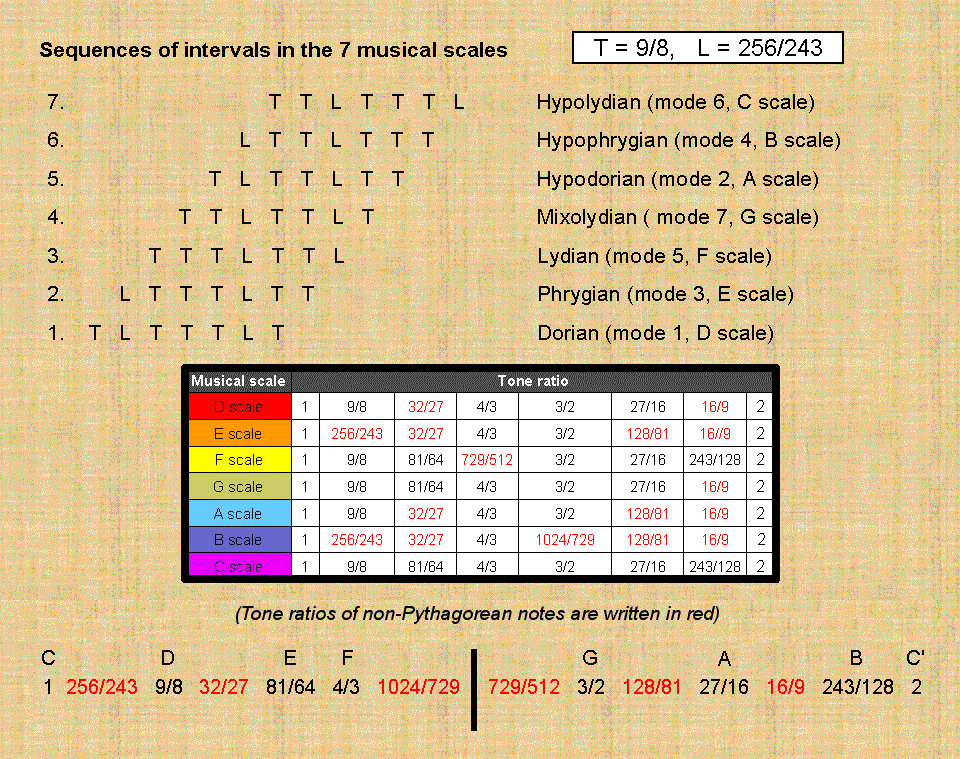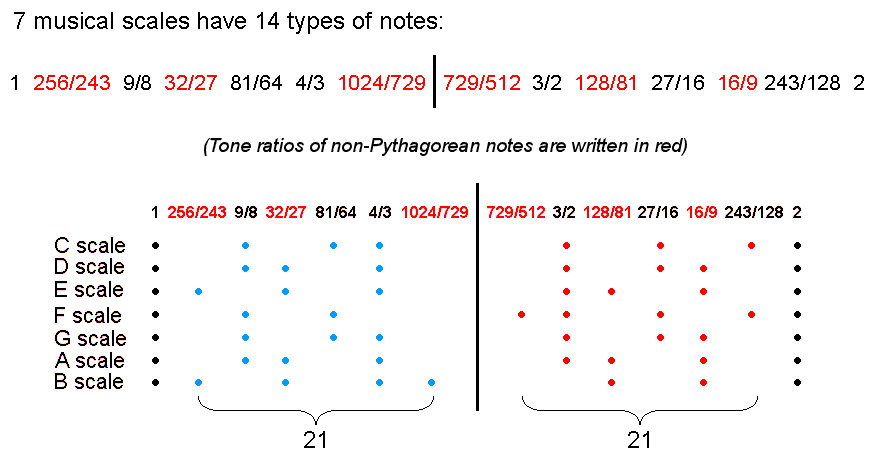They comprise 26 Pythagorean notes made up of 14 notes (denoted below by blue dots) up to, and including, the perfect fourth) & 12 inversions (denoted by light red dots), as well as 16 non-Pythagorean notes denoted by grey dots: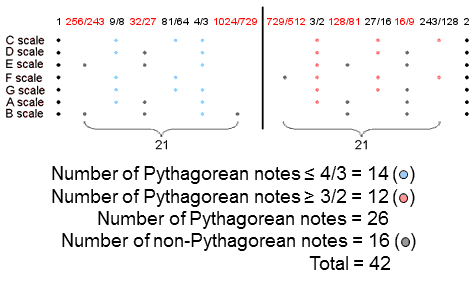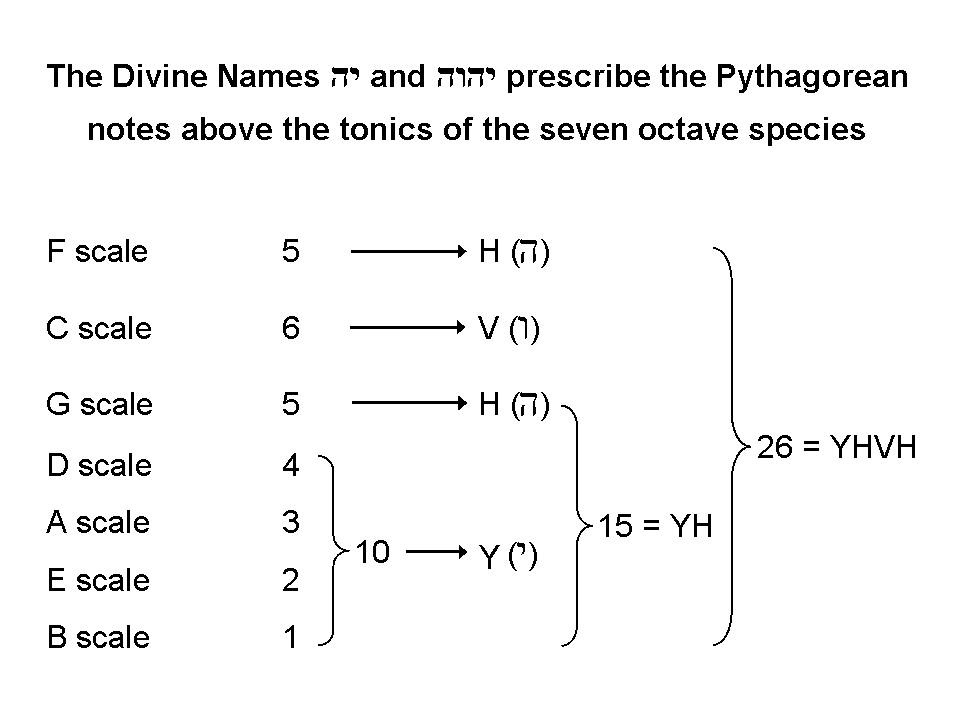Amazingly, the letter values of the Divine Name YAHWEH, which prescribes how many Pythagorean notes make up the seven octave species, quantify the Pythagorean notes in subsets of these species.

The 26:16 division of Pythagorean and non-Pythagorean notes and the 14:12 division in the 26 Pythagorean notes and their inversions manifest in sacred geometries as well. Article 4 shows how the Godnames of the 10 Sephiroth mathematically prescribe the first 6 enfolded polygons as a holistic structure. For example, they have 26 corners, both sets of the first 6 polygons having 50 corners, where 26 is the number of YAHWEH, the Godname of Chokmah, and 50 is the number of ELOHIM, the Godname of Binah, which follows Chokmah in the Tree of Life. The last two enfolded polygons (octagon & decagon) have 14 corners outside the root edge, the first four enfolded polygons having 12 corners. Other combinations of polygons also display this division. Which combination is the "correct" one is irrelevant to the present discussion. The point is that this division exists naturally in the six polygons. Its counterpart in the Sri Yantra is just as natural. The nine parent triangles that generate the 42 triangles through their overlap have 27 corners of which one is the nadir of the central triangle and 26 are outermost corners of these 42 triangles. They comprise the 14 of the 15 light blue corners of the five downward-pointing triangles with blue sides representing the Shakti ("feminine") aspect of creation and the 12 light red corners of the four upward-pointing triangles with red sides that represent the Siva ("masculine") aspect. The 12 Pythagorean notes that span the 4/3 interval between the perfect fifth and the octave therefore correspond to the Siva principle, whilst the 14 Pythagorean notes spanning the 4/3 interval between the perfect fourth and the tonic correspond to the Shakti principle. This is consistent with the octave representing the complete domain that is divided up into the eight notes, for the undivided whole before division is always masculine in the metaphysical sense, whilst the whole patterned into parts is always feminine. In the Tree of Life, the point of Kether transforms into the amorphous line of Chokmah (the "Great Father") and then into the simplest shape – the triangle of the Supernal Triad, completed by Binah (the "Great Mother") as its third corner.

The 8 Church modes
The 21:21 division of the 42 notes between the tonic and octave of the seven diatonic scales is only part of the general divisions displayed by holistic systems, namely,

384 = 192 + 192,

where

192 = 24 + 168,
24 = 3 + 21

and

168 = 84 + 84,

(see here). So what is the set of 24 notes that includes the 21 notes (and likewise for their inversions)? It is the 24 notes (and their 24 inversions) between the tonic and octave of the eight musical modes that have been the basis of the music of the Roman Catholic Church for hundreds of years. The eight modes consist of the seven diatonic scales and a repetition of the Dorian mode (D scale) known as the Hypomixolydian mode, which differs in what notes are the finalis (ending note for a musical composition) and the dominant (reciting note) (see #25). The six notes between the tonic and octave of the Dorian mode consist of four Pythagorean notes and two non-Pythagorean notes, so that the eight modes have (42+6=48) notes between tonic and octave that comprise (26+4=30) Pythagorean notes (16 up to the perfect fourth and 14 inversions) and (16+2=18) non-Pythagorean notes: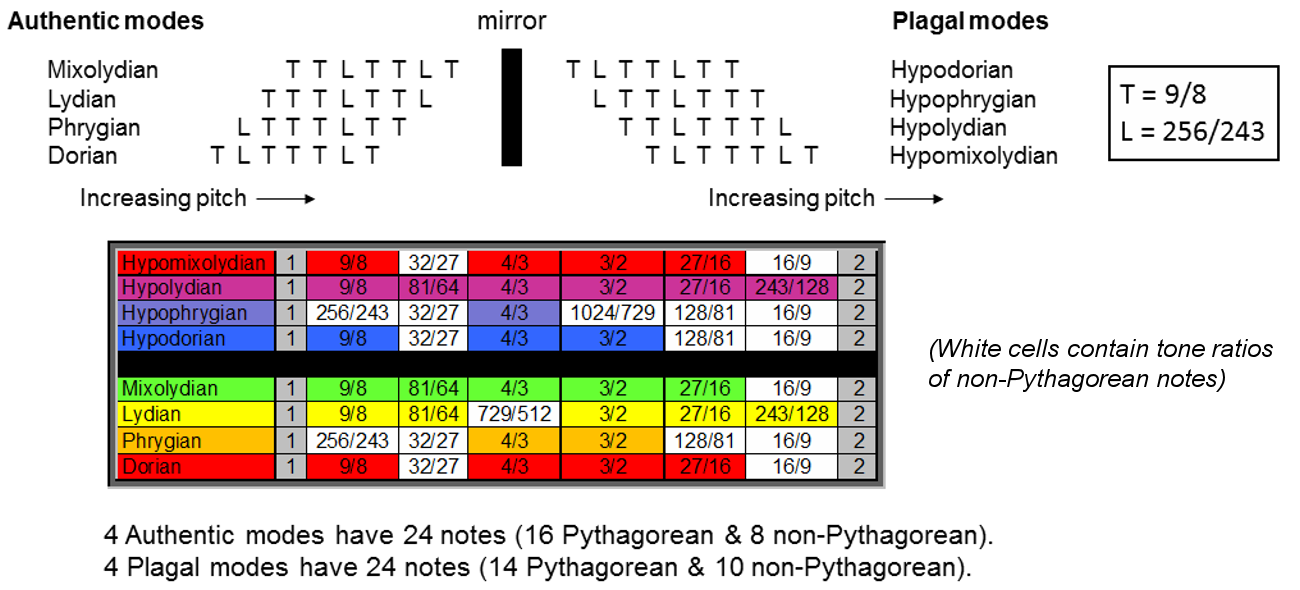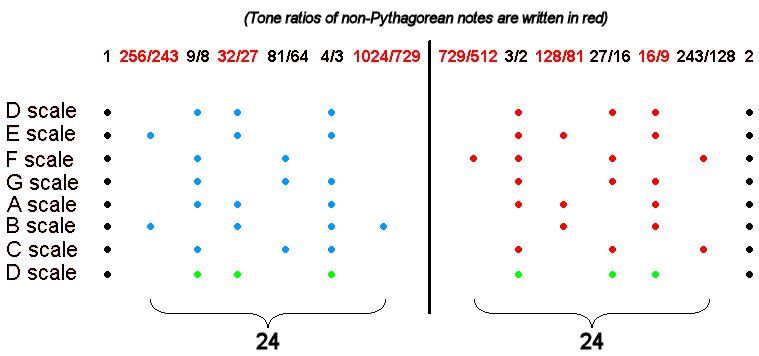The six green dots denote the six notes between the tonic and octave of the Hypomixolydian mode. Notice that its three notes up to the perfect fourth (the "3" in the set of 24 notes) comprise two Pythagorean notes and one non-Pythagorean note. Similarly, the three inversions comprise two Pythagorean notes and one non-Pythagorean note. It is readily verified by inspection of the table above that the Dorian mode is unique in being the only one of the seven diatonic scales that has three notes and three inversions, each set of which comprises two Pythagorean and one non-Pythagorean note. The significance of this will emerge shortly. The Dorian mode (and, of course, the identical Hypomixolydian mode) is also unique in being the only mode whose sequence of intervals: TLTTTLT is its own mirror image. The significance of this will also become apparent shortly.

The 48 notes between the tonic and octave of the eight Church modes comprise 24 notes with tone ratios up to 1024/729 and their 24 inversions. 48 is the number value of Kokab, the Mundane Chakra of Hod. That this parameter of the eight modes is a Kabbalistic number is an indication that they constitute a holistic system. Its 24:24 division is characteristic of such systems because it is a component of their general divisions, as discussed above. The four "authentic" modes: Dorian, Phrygian, Lydian & Mixolydian have sequences of tone intervals that are the mirror images of the sequences in their counterpart plagal modes. This means that the (18+18=36) notes in three pairs of modes and their mirror images can be assigned to 36 points on three great circles spaced over a sphere 120° apart,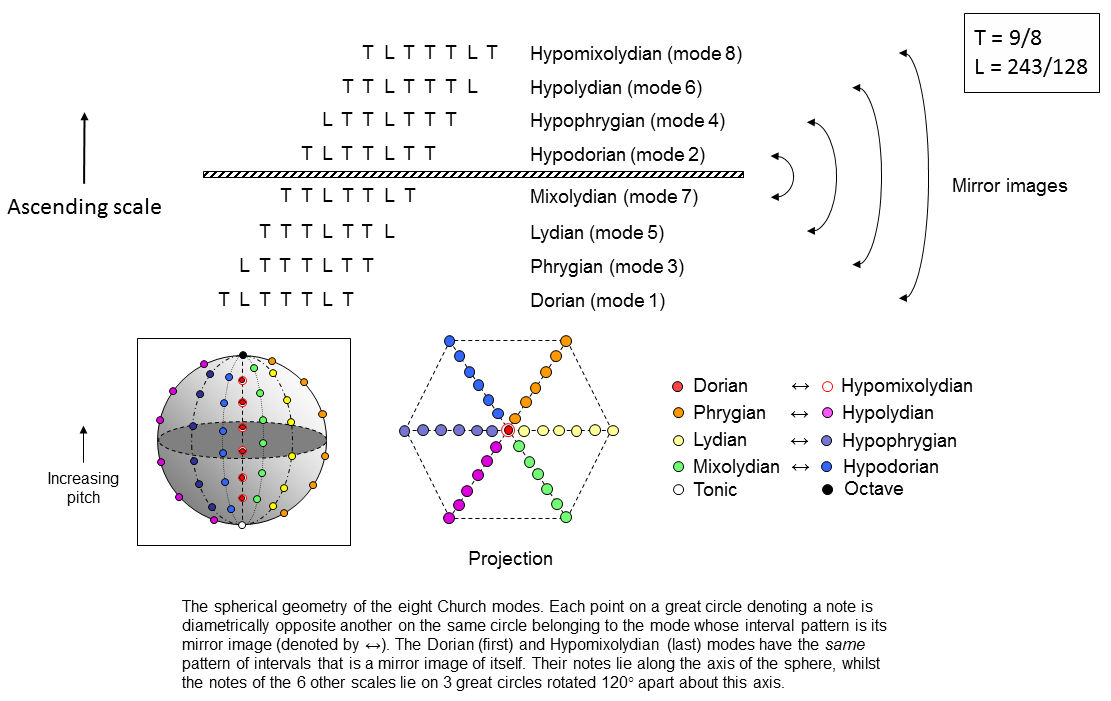with the 12 notes of the two identical modes assigned to six points arranged along its axis, its North Pole denoting the octave and its South Pole denoting the tonic. Notes represented by points in the upper (northern) hemisphere are the inversions of the notes denoted by points in the lower (southern) hemisphere that are their mirror images. The projection of these points onto the plane of the equator forms the six arms of a starfish shape, the centre of this hexagonal pattern representing the axis of the sphere formed by the 14 notes of the identical Dorian and Hypomixolydian modes.

Alternatively, the 48 notes may be assigned to four great circles spaced 45° apart. In this representation, no notes are arranged along the axis, so that the sense of the Dorian mode as the starting point, or generator, of the seven diatonic scales is missing. In either case, however, a minimal set of 50 points is sufficient to mark out all the notes of the eight musical modes. It illustrates the archetypal nature of ELOHIM, the Godname of Binah with number value 50. As the octave belongs to the Pythagorean scale, there are 49 notes above the tonic that include (1+30=31) Pythagorean notes and the 36 notes in the six modes whose interval sequences are not mirror images of themselves. This shows how:

1. EL CHAI, the Godname of Yesod with number value 49,
2. EL, the Godname of Chesed with number value 31,
3. ELOHA, the Godname of Geburah with number value 36,

prescribe a spherical distribution of the notes that make up the eight Church modes.

The number value 18 of CHAI is the number of their non-Pythagorean notes. The letter values of EL are E = 1 and L = 30. They denote the octave and the 30 Pythagorean notes in the eight musical modes. EHYEH, the Godname of Kether with number value 21, prescribes the 21 notes and their 21 inversions between the tonic and octave of the seven diatonic scales. YAHWEH, the Godname of Chokmah with number value 26, measures how many of these notes are Pythagorean. It also prescribes the 21 Pythagorean notes (including the octave) that lie on the three great circles representing the six diatonic scales whose sequences of successive intervals are not mirror images of themselves.

The heptagon expresses the seven diatonic scales when constructed from tetractyses because the 42 yods surrounding its centre symbolise their 42 notes: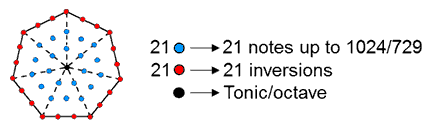The 21 internal, blue yods denote the 21 notes with tone ratios up to 1024/729 and the 21 red yods on its boundary denote their 21 inversions, the centre of the heptagon denoting both the tonic and the octave. They are prescribed by EHYEH (AHIH), the Godname of Kether: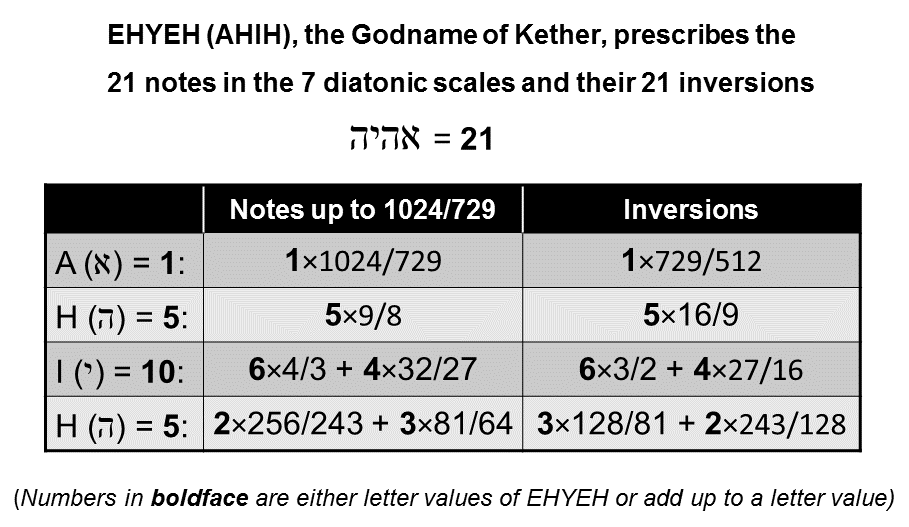The eight trigrams whose 64 pairings generate the 64 hexagrams used in the ancient divinatory system known as I Ching are generated in a way that is analogous to the successive formation of the eight Church modes: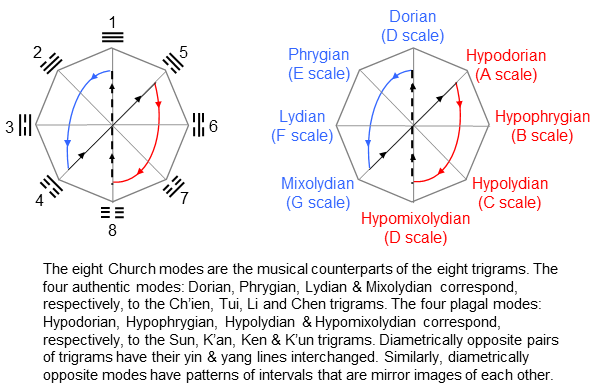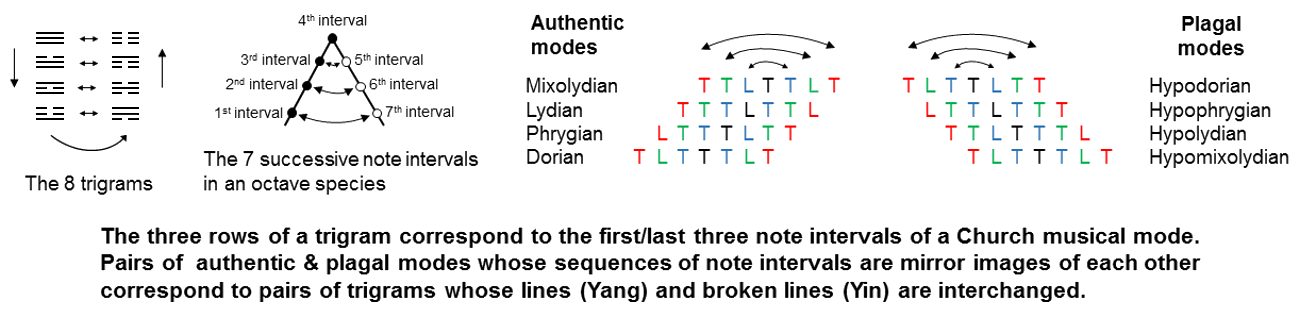As we move from the maximum Yang of trigram 1 (Heaven) towards the maximum Yin of trigram 8 (Earth), so the modes shift towards the crossover between notes (representing the "male" domain of the scale) and their inversions (representing the "female" domain). The polar opposite of a trigram is one with Yang lines and Yin lines interchanged. Similarly, each Authentic Mode has a Plagal Mode as the mirror image of its sequence of tone intervals (T) and leimmas (L). The fourth interval (the interval between the fourth note and the fifth note) acts as a mirror, reflecting the first, second & third intervals so that they become, respectively, the seventh, sixth & fifth intervals of the plagal counterpart of the Authentic Mode, whilst reflecting the fifth, sixth & seventh intervals so that they become, respectively, the third, second and first intervals of the plagal counterpart. Either set of three intervals corresponds to the three rows of a trigram. Just as the cycle of generation of trigrams ends at the eight one, so the generation of diatonic scales, starting at successive notes of the Dorian Mode, ends at the eighth one (the Hypomixolydian mode), which, as a sequence of intervals, is identical to the first mode.

Below are the ways in which four sacred geometries represent the notes in either the seven diatonic scales or the eight Church modes.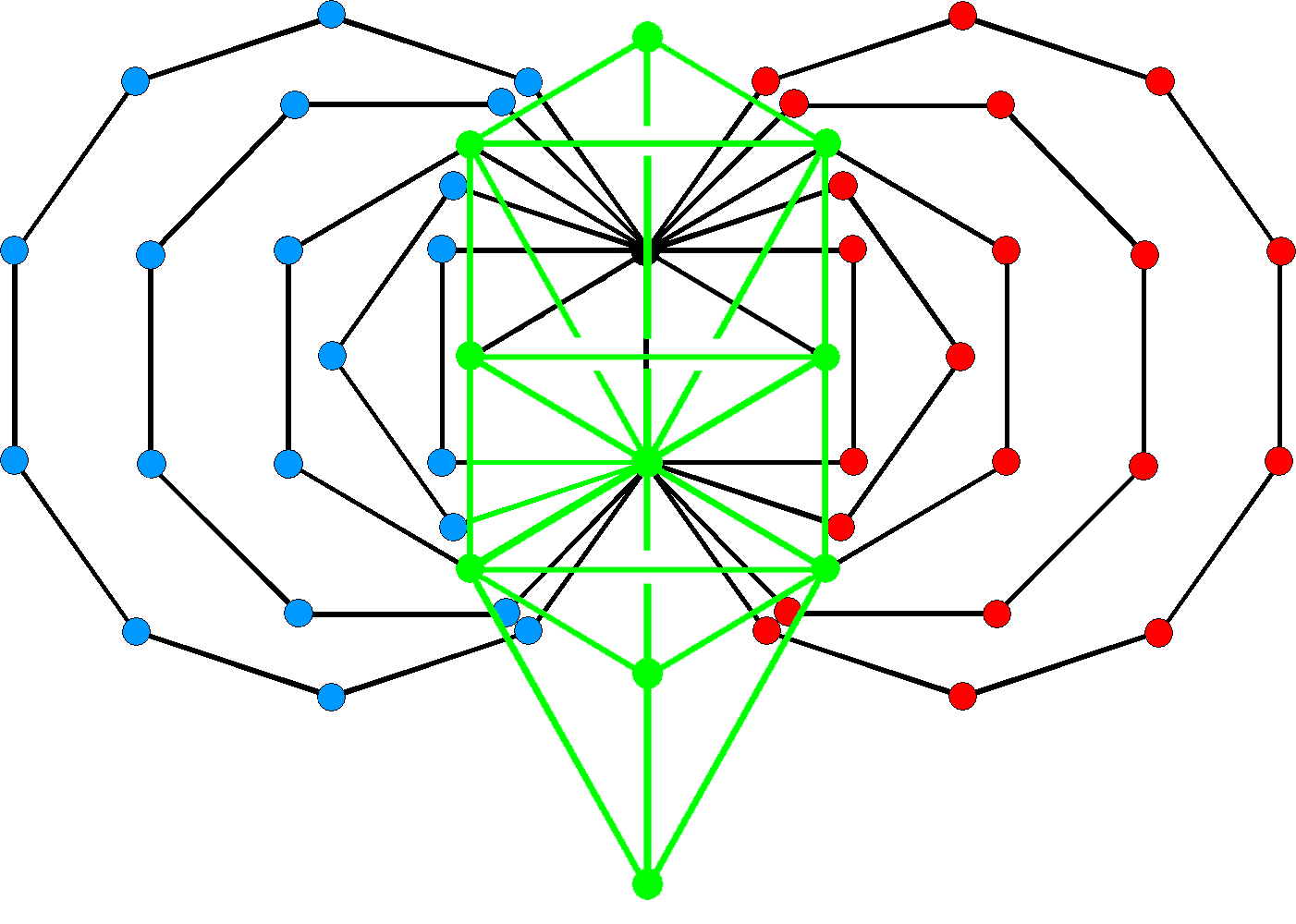21 red corners & 21 blue corners of the first (6+6) enfolded polygons are intrinsic to them. The inner Tree of Life consists of (7+7) enfolded, regular polygons with 70 corners. As the dodecagon (the last of these types of polygons) has 10 corners outside the shared root edge, the first (6+6) enfolded polygons have 50 corners. The 7 polygons on the right have 24 corners outside the root edge, as do the 7 polygons on the left. Of these corners, three coloured green coincide with the positions of Sephiroth in the outer Tree of Life. They are the corner of the triangle (Chesed & Geburah), the tops of the two hexagons (Chokmah & Binah) and their lowest corners (Netzach & Hod). This means that 21 red corners and 21 blue corners are intrinsic to the inner Tree of Life. They correspond to the 21 notes and their 21 inversions between the tonic and octave of the 7 diatonic scales. The (3+3) green corners denote the 2nd, 3rd & 4th notes in the Hypomixolydian mode and their inversions. These 6 notes are identical to the six notes between the tonic and octave of the Dorian mode because these two modes have the same pattern of note intervals. We see that corners shared by the outer and inner Trees of Life denote the notes of the eight modes, whilst corners intrinsic to the inner Tree of Life denote the notes of the seven distinct octave species. As pointed out above, each set of three notes consists of two Pythagorean notes and one non-Pythagorean note. This corresponds to how the top and bottom corners of the hexagons coincide with Sephiroth on either side pillar that are shared between adjacent Trees of Life, whilst the third corner (the corner of the triangle) coincides with Chesed or Geburah, both of which belong only to one Tree. The endpoints of the root edge denote the tonic and octave common to all the scales. They correspond to the North and South Poles of the spherical representation of the eight Church modes, which was discussed earlier.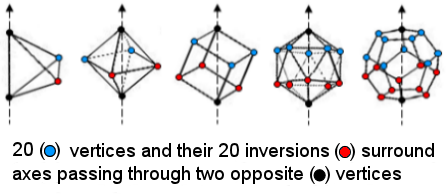The 5 Platonic solids have 50 vertices. Forty vertices surround axes drawn through two opposite vertices, shown as black. If the 5 regular polyhedra are considered nested in one another so that their axes coincide and their sizes are adjusted so that all their uppermost vertices coincide and all their lowest vertices coincide, the nest now has 42 distinct vertices. Their upper halves have 20 blue vertices, sharing one black vertex; their lower halves have 20 red vertices and share one black vertex. The 21:21 division characteristic of holistic systems appears in the 5 Platonic solids as the 21:21 division in the 42 points in 3-d space minimally required to fit them inside one another so that only a pair of vertices in each polyhedron occupies the same two points in space.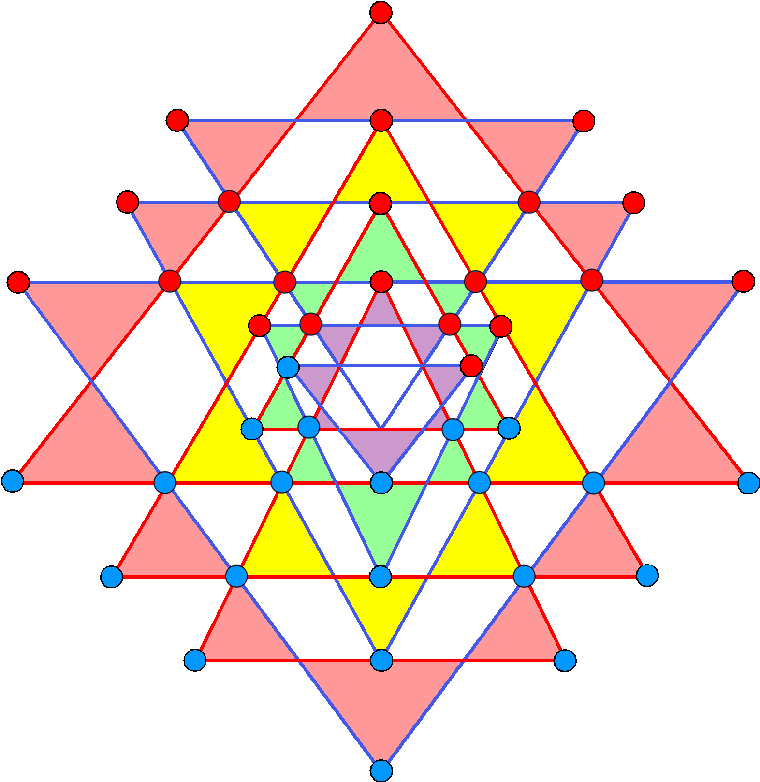Sri Yantra 42 triangles in the Sri Yantra surround its central (white) triangle. They have 21 blue, outward-pointing tips in one half of the Sri Yantra and 21 red tips in its other half. They denote the 21 notes between the tonic and octave of the 7 diatonic scales and their 21 inversions.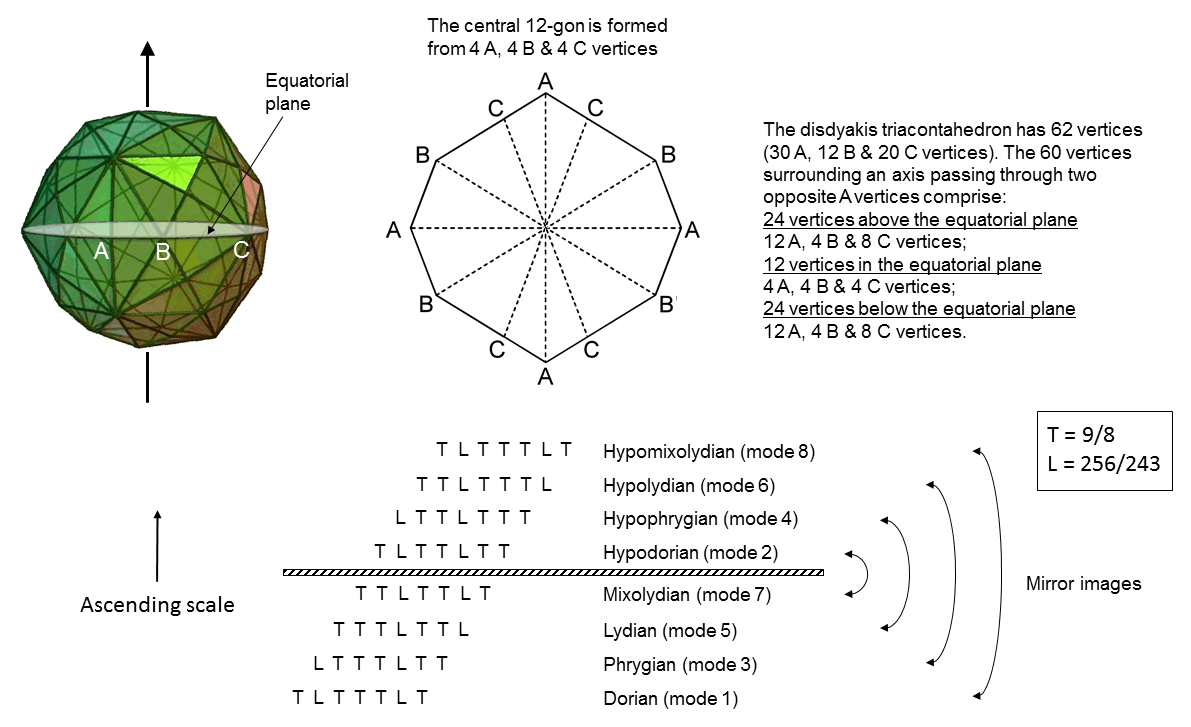The disdyakis triacontahedron has 62 vertices. They are of three types: A (×30), B (×12) and C (×20). Considering the straight line passing through two opposite A vertices as its axis, 60 vertices (28 A, 12 B & 20 C) surround it. There are 12 vertices (4 A, 4 B & 4 C) in the equatorial plane that form the corners of a 12-gon. 48 vertices surround the axis above and below this plane. Each half has 24 vertices that comprise 12 A, 4 B & 8 C vertices. Including the vertices at the top and bottom, the 50 vertices not lying in the equatorial plane are the counterpart of both the 50 notes in the spherical representation of the 8 Church modes (see above) and the 50 corners of the first (6+6) enfolded polygons of the inner Tree of Life. They are prescribed by ELOHIM, the Godname of Binah with number value 50. The A vertices at the top and bottom of the disdyakis triacontahedron denote the tonic and octave, whilst the 24 vertices in each half denote the 24 notes in the 8 Church musical modes and their 24 inversions.

 << Previous    1...   40  41    43  44    Next >>

Home